# Wiener space, abstract

The probability distribution of a Brownian motion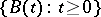is a Gaussian measure (cf. also Constructive quantum field theory) that can be supported by the spaceof continuous functions. For this reason,is also called the classical Wiener space. This notion can be generalized to a Banach space on which a Gaussian measure can be introduced.

Advanced stochastic analysis can be carried out on a Wiener space. The analysis was initiated by P. Lévy and N. Wiener, and a systematic development was made by R.H. Cameron and W.T. Martin, I.E. Segal, L. Gross, K. Itô, and others.

Following [a2], one can introduce the notion of an abstract Wiener space, which is a generalization of the classical Wiener space.

Letbe a real separable Hilbert space with norm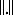. Onone introduces the weak Gaussian distributionin such a way that on any finite-, say-, dimensional subspaceofthe restriction oftois the-dimensional standard Gaussian distribution. In fact,may be called the weak white noise measure. A semi-norm (or norm)onis called a measurable norm if for any positivethere exists a finite-dimensional projection operator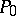such that for any finite-dimensional projection operatororthogonal tothe inequalityholds.

Now, let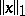be a measurable norm onand letbe the completion ofwith respect to this norm (cf. Complete space). Thenis a Banach space. Letbe the-ring generated by the cylinder subsets of(cf. Cylinder set). For a cylinder set measureon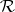induced by the Gaussian distribution on, the measureis countably additive on. Therefore, taking the-fieldgenerated by, a measure space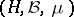is obtained.

The classical definition of an abstract Wiener space is given as follows. First, take a Hilbert spacewith normand take a measurable norm, to obtain a Banach space. The injection mapping fromintois denoted by. Then the triple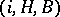is called an abstract Wiener space. This means that a weak measure oncan be extended to a completely additive measure supported by. A stochastic analysis can be developed for this latter measure (see [a4]).

One of the developments of the notion of an abstract Wiener space is that of a rigged Hilbert space, due to I.M. Gel'fand and N.Ya. Vilenkin (see [a1]). Letbe a real Hilbert space and letbe a countably Hilbert nuclear space that is continuously imbedded in. The dual spaceofgives rise to the rigged Hilbert space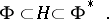Given a characteristic functional,, that is,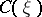is continuous in, positive definite and, there exists a countably additive probability measurein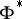such that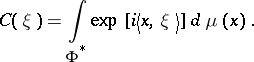A typical example of a rigged Hilbert space is the triple consisting of the Hilbert space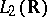, the Schwartz spaceand the spaceof tempered distributions (cf. Generalized function). White noise is also an important example ofon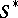; it has characteristic functional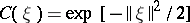. The analysis on the function space with the white noise measure is well developed (see [a3]).